# 卢卡斯定理（十分钟带你看懂）

1.乘法逆元

2.费马小定理：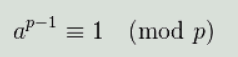3.扩展欧几里得

———————————————————————————————————————————–

———————————————————————————————————————————–

———————————————————————————————————————————–

12 / 4 mod 7 = ? 很显然结果是3

F / A mod C = ?

(1) 模方程 A * X = 1(mod C) 存在解
(2) A | F (F % A == 0)

14=5*2+4
5=4+1

1=5-4=5-(14-5*2)=5*3-14

———————————————————————————————————————————–

Lucas定理针对该取值范围较大又不太大的情况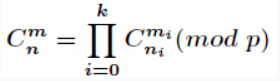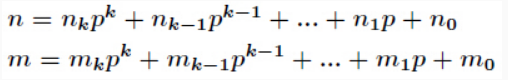已知C(n, m) mod p = n!/(m!(n - m)!) mod p。当我们要求（a/b）mod p的值，且b很大，无法直接求得a/b的值时，我们可以转而使用乘法逆元k，将a乘上k再模p，即(a*k) mod p。 其结果与(a/b) mod p等价。
下面附上Lucas定理的一种证明，见下图，参考冯志刚《初等数论》第37页。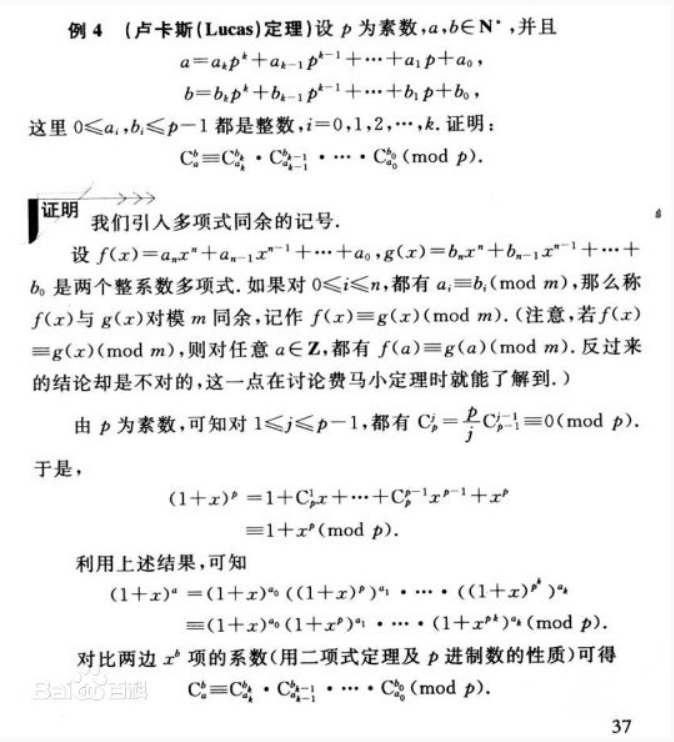对于该定理的理解，也很好理解：
其实大可不必看中间一大堆文字描述，直接看下面公式的推导：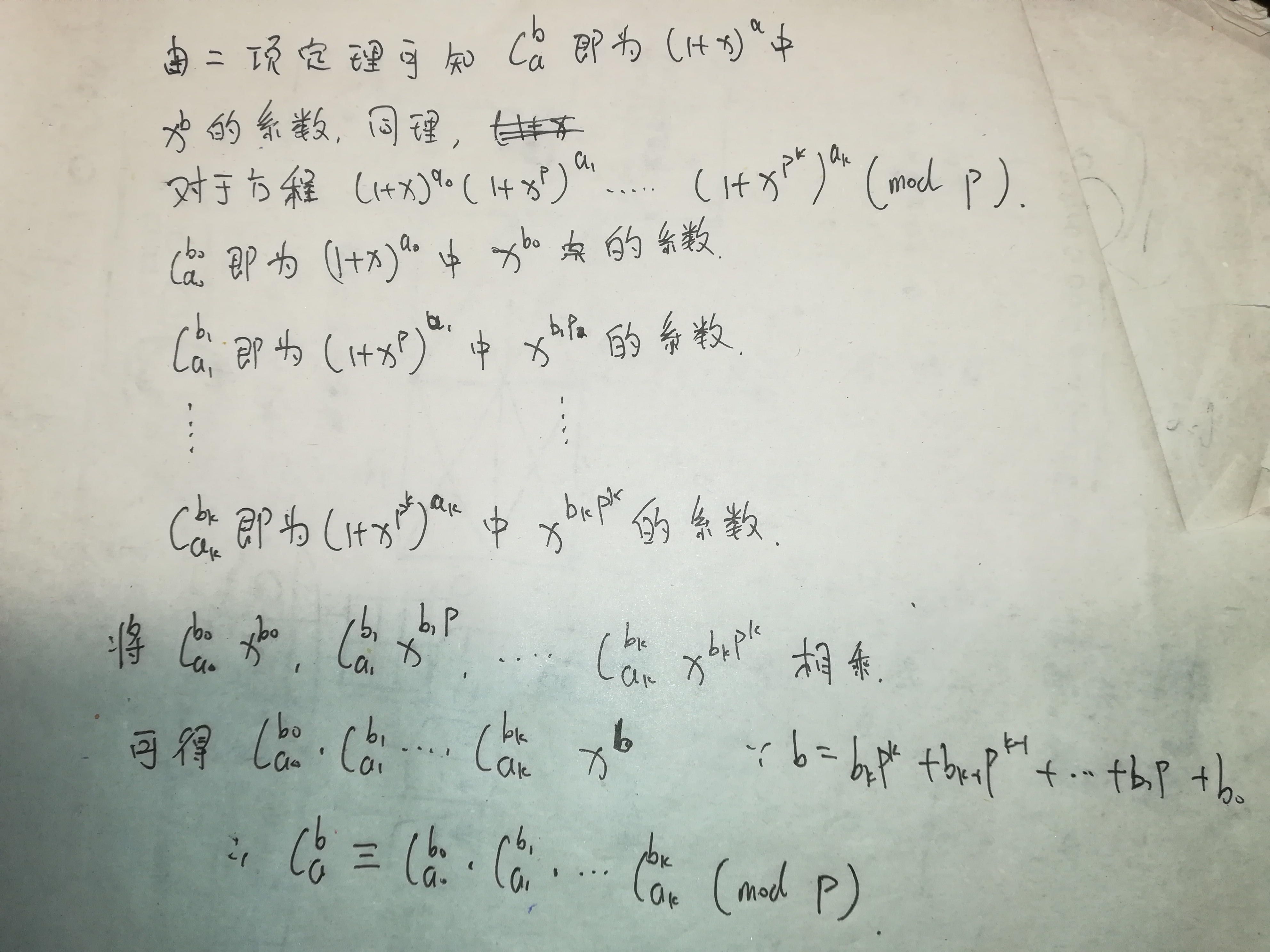———————————————————————————————————————————–
代码实现：
费马小定理实现（其实跟拓展gcd没多大区别，换了个公式而已）

#include <iostream>
#include <stdio.h>
#include <algorithm>
#include<cstring>

using namespace std;

typedef long long ll;

ll mulit(ll a,ll b,ll m){
ll ans=0;
while(b){
if(b&1) ans=(ans+a)%m;
a=(a<<1)%m;
b>>=1;
}
return ans;
}

ll quick_mod(ll a,ll b,ll m){
ll ans=1;
while(b){
if(b&1){
ans=mulit(ans,a,m);
}
a=mulit(a,a,m);
b>>=1;
}
return ans;
}

ll comp(ll a,ll b,ll m){
if(a<b) return 0;
if(a==b) return 1;
if(b>a-b) b=a-b;
ll ans=1,ca=1,cb=1;
for(int i=0;i<b;i++){
ca=ca*(a-i)%m;
cb=cb*(b-i)%m;
}
ans=ca*quick_mod(cb,m-2,m)%m;
return ans;
}

ll lucas(ll a,ll b,ll m){
ll ans=1;
while(a&&b){
ans=(ans*comp(a%m,b%m,m))%m;
a/=m;
b/=m;
}
return ans;
}

int main()
{
ll a,b,m;
while(cin>>a>>b>>m){
cout<<lucas(a,b,m)<<endl;
}
return 0;
}



#include <iostream>
#include <stdio.h>
#include <algorithm>
#include<cstring>

using namespace std;

typedef long long ll;

ll exgcd(ll a,ll b,ll& x,ll& y){
if(a%b==0){
x=0,y=1;
return b;
}
ll r,tx,ty;
r=exgcd(b,a%b,tx,ty);
x=ty;
y=tx-a/b*ty;
}

ll comp(ll a,ll b,ll m){
if(a<b) return 0;
if(a==b) return 1;
if(b>a-b) b=a-b;
ll ans=1,ca=1,cb=1;
for(int i=0;i<b;i++){
ca=ca*(a-i)%m;
cb=cb*(b-i)%m;
}
ll x,y;
exgcd(cb,m,x,y);
x=(x%m+m)%m;
ans=ca*x%m;
return ans;
}

ll lucas(ll a,ll b,ll m){
ll ans=1;
while(a&&b){
ans=(ans*comp(a%m,b%m,m))%m;
a/=m;
b/=m;
}
return ans;
}

int main()
{
ll a,b,m;
int n;
cin>>n;
while(n--){
cin>>a>>b>>m;
cout<<lucas(a+b,b,m)<<endl;
}
return 0;
}